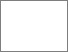## First observation of the decay $\Lambda_b^0 \rightarrow \Lambda_c^+ \bar{D}^{(\ast)0} K^-$ in preparation of a pentaquark search in the $\Lambda_c^+ \bar{D}^{(\ast)0}$ system at the LHCb experiment

Stahl, MarianPreview
PDF, English - main document
This thesis reports the first observation of the decays $\Lambda_b^0 \rightarrow \Lambda_c^+ \bar{D}^0 K^-$ and $\Lambda_b^0 \rightarrow \Lambda_c^+ \bar{D}^\ast(2007)^0 K^-$ using data corresponding to an integrated luminosity of 3 fb${}^-1$ collected at 7 and 8 TeV center-of-mass energies in proton-proton collisions with the LHCb detector. A future amplitude analysis of these $\Lambda_b^0$ decays allows to search the $\Lambda_c^+ \bar{D}^{(\ast)0}$ system for resonant contributions from $P_c^+$ pentaquarks. Two of which have been discovered in 2015 by the LHCb experiment in the J/$\psi p$ system. Observing $P_c^+$ pentaquarks in the $\Lambda_c^+ \bar{D}^{(\ast)0}$ system would allow for further insight to the nature of these resonances.
In addition, the measurement of the $\Lambda_b^0 \rightarrow \Lambda_c^+ \bar{D}^0 K^-$ and $\Lambda_b^0 \rightarrow \Lambda_c^+ \bar{D}^\ast(2007)^0 K^-$ branching fractions relative to the decay $\Lb\to\Lc\Dsm$ is made, resulting in \begin{alignat}{1} \frac{\mathscr{B}(\Lambda_b^0 \rightarrow \Lambda_c^+ \bar{D}^0 K^-)}{\mathscr{B}(\Lambda_b^0 \rightarrow \Lambda_c^+ D_s^-)} &= \left(14.04 \pm 0.58 \pm 0.33 \pm 0.45 \right)\,\% \nonumber \\ \frac{\mathscr{B}(\Lambda_b^0 \rightarrow \Lambda_c^+ \bar{D}^\ast(2007)^0 K^-)}{\mathscr{B}(\Lambda_b^0 \rightarrow \Lambda_c^+ D_s^-)} &= \left(43.5 \pm 1.4 {}^{+1.2}_{-0.8} \pm 1.4 \right)\,\% \nonumber \end{alignat} The first uncertainty is statistical, the second systematic and the third is due to the knowledge of the branching fractions of $D_s^- \rightarrow K^- K^+ \pi^-$ and $\bar{D}^0 \rightarrow K^+ \pi^-$. It is shown that the obtained result agrees with previously measured $B\rightarrow D\bar{D}^{(\ast)0} K^-$ branching fractions, as expected from the lowest order approximation of QCD factorisation.
The developed methods are integral components of the future amplitude analysis of $\LbLcDzbstK$. These and further algorithms presented here were developed in a modularised way to be applied to a variety of analyses at \lhcb, in particular pentaquark searches.